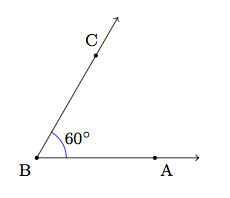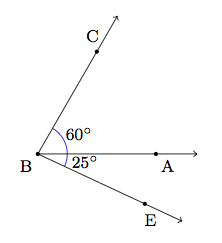# Measuring Angles

Alignments to Content Standards: 4.MD.C.7 4.MD.C.6 4.G.A.1

1. Draw an angle that measures 60 degrees like the one shown here:2. Draw another angle that measures 25 degrees. It should have the same vertex and share side $\overrightarrow{BA}$.
3. How many angles are there in the figure you drew? What are their measures?
4. Make a copy of your 60 degree angle. Draw a different angle that measures 25 degrees and has the same vertex and also shares side $\overrightarrow{BA}$.
5. How many angles are there in the figure you drew? What are their measures?

## IM Commentary

The purpose of this task is to gain experience drawing and measuring angles, developing an understanding of the additive structure of angles. This task gives good practice with MP.5, Use Appropriate Tools Strategically, as a protractor should be used to construct the angles. Since the protractor is not an exact instrument, students will not necessarily obtain the mathematically derived answers in the solution (35 degrees and 85 degrees). They should, however, find answers very close to these and, with their understanding of the additive nature of angles, know what the exact answer should be even if their drawings or measurements were not exact.

This task was adapted from problem #4 on the 2012 American Mathematics Competition (AMC) 10A Test. Observers might be surprised that a task that was historically considered to be appropriate for a high school mathematics competition aligns to an elementary standard in the Common Core. While the original statement of the problem required more sophisticated visualization skills than the current version, the mathematical ideas the original problem addressed are accessible to 4th graders in an instructional situation. This earlier introduction to angles and angle measure brings states who have adopted the Common Core in line with other high-achieving countries.

The numbers in the original problem were changed from 24 and 20 to 60 and 25 so that the pictures are easier to draw and interpret: also the exam question asked students to picture the construction without a drawing for reference and then to identify the smaller of the two possible angle measures. For the 2012 AMC 10A, which was taken by 73,703 students, the multiple choice answers for the problem had the following distribution:

 Choice Answer Percentage of Answers (A) 0 1.85 (B) 2 3.19 (C)* 4 78.33 (D) 6 2.85 (E) 12 1.81 Omit -- 11.95

Of the 73,703 students: 36,206 or 49% were in 10th grade, 25,498 or 35% were in 9th grade, and the remainder were below than 9th grade.

## Solution

1. Here is an angle, labelled $\angle ABC$, whose measure is 60$^\circ$:2. Here is a picture of an angle, labelled $\angle ABD$, measuring 25$^\circ$ and sharing $\overrightarrow{BA}$ with $\angle ABC$:3. The picture above has three angles. There is the original $\angle ABC$ which measures 60$^\circ$. There is $\angle ABD$ which we added and it measures 25$^\circ$. The third angle, $\angle CBD$, makes up $\angle ABC$ together with $\angle ABD$. This means that $$\angle CBD + \angle ABD = \angle ABC.$$ We know that $\angle ABD = 25^\circ$ and $\angle ABC = 60^\circ$ so $$\angle CBD = 60^\circ - 25^\circ = 35^\circ.$$

4. We can also make a 25$^\circ$ angle with ray $BA$ as pictured below (we labelled the fourth point $E$ to distinguish it from the point $D$ in the previous picture):5. In this picture we again have $\angle ABC$ whose measure is 60$^\circ$. We have $\angle ABE$ whose measure is 25$^\circ$. Finally, there is $\angle CBE$ which is made up of $\angle ABE$ and $\angle ABC$ so $$\angle CBE = 25^\circ + 60^\circ = 85^\circ.$$